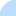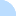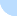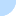## DebcheckDebcheck - buster  Summary  All packages (light) main only  Broken PreDepends  Broken Depends  Broken Recommends  Broken Suggests  HalfBroken PreDepends  HalfBroken Depends  HalfBroken Recommends  HalfBroken Suggests  Build-Depends  HalfBroken Build-Depends  Malformed Build-Depends  OldLibs  Priority  Old Standards-Version  No Standards-Version  Wrong Standards-Version Syntax other  Depends Main on !Main all sections  Broken PreDepends  Broken Depends  Broken Recommends  Broken Suggests  HalfBroken PreDepends  HalfBroken Depends  HalfBroken Recommends  HalfBroken Suggests  Build-Depends  HalfBroken Build-Depends  Malformed Build-Depends  OldLibs  Priority  Old Standards-Version  No Standards-Version  Wrong Standards-Version SyntaxDistribution: sid bullseye buster stretch

# Problems for source package snort

Current Source Version: 2.9.7.0-5
Current Maintainer: Javier Fernández-Sanguino Peña <jfs@debian.org>
Section: main
BTS entry: https://bugs.debian.org/src:snort

# Binary Package: snort-rules-default (Version: 2.9.7.0-5)

BTS entry: https://bugs.debian.org/snort-rules-default

### Half Broken Relationships

(not necessarily bugs)

Package has a Suggests on snort-mysql (>= 2.2.0) which cannot be satisfied on armhf. At least one of snort (>= 2.2.0) | snort-pgsql (>= 2.2.0) | snort-mysql (>= 2.2.0) can be satisfied however.
Package has a Suggests on snort-mysql (>= 2.2.0) which cannot be satisfied on arm64. At least one of snort (>= 2.2.0) | snort-pgsql (>= 2.2.0) | snort-mysql (>= 2.2.0) can be satisfied however.
Package has a Suggests on snort-mysql (>= 2.2.0) which cannot be satisfied on armel. At least one of snort (>= 2.2.0) | snort-pgsql (>= 2.2.0) | snort-mysql (>= 2.2.0) can be satisfied however.
Package has a Suggests on snort-mysql (>= 2.2.0) which cannot be satisfied on s390x. At least one of snort (>= 2.2.0) | snort-pgsql (>= 2.2.0) | snort-mysql (>= 2.2.0) can be satisfied however.
Package has a Suggests on snort-mysql (>= 2.2.0) which cannot be satisfied on amd64. At least one of snort (>= 2.2.0) | snort-pgsql (>= 2.2.0) | snort-mysql (>= 2.2.0) can be satisfied however.
Package has a Suggests on snort-mysql (>= 2.2.0) which cannot be satisfied on ppc64el. At least one of snort (>= 2.2.0) | snort-pgsql (>= 2.2.0) | snort-mysql (>= 2.2.0) can be satisfied however.
Package has a Suggests on snort-mysql (>= 2.2.0) which cannot be satisfied on mipsel. At least one of snort (>= 2.2.0) | snort-pgsql (>= 2.2.0) | snort-mysql (>= 2.2.0) can be satisfied however.
Package has a Suggests on snort-mysql (>= 2.2.0) which cannot be satisfied on i386. At least one of snort (>= 2.2.0) | snort-pgsql (>= 2.2.0) | snort-mysql (>= 2.2.0) can be satisfied however.
Package has a Suggests on snort-mysql (>= 2.2.0) which cannot be satisfied on mips. At least one of snort (>= 2.2.0) | snort-pgsql (>= 2.2.0) | snort-mysql (>= 2.2.0) can be satisfied however.
Package has a Suggests on snort-mysql (>= 2.2.0) which cannot be satisfied on mips64el. At least one of snort (>= 2.2.0) | snort-pgsql (>= 2.2.0) | snort-mysql (>= 2.2.0) can be satisfied however.
Package has a Suggests on snort-pgsql (>= 2.2.0) which cannot be satisfied on mips64el. At least one of snort (>= 2.2.0) | snort-pgsql (>= 2.2.0) | snort-mysql (>= 2.2.0) can be satisfied however.
Package has a Suggests on snort-pgsql (>= 2.2.0) which cannot be satisfied on mips. At least one of snort (>= 2.2.0) | snort-pgsql (>= 2.2.0) | snort-mysql (>= 2.2.0) can be satisfied however.
Package has a Suggests on snort-pgsql (>= 2.2.0) which cannot be satisfied on mipsel. At least one of snort (>= 2.2.0) | snort-pgsql (>= 2.2.0) | snort-mysql (>= 2.2.0) can be satisfied however.
Package has a Suggests on snort-pgsql (>= 2.2.0) which cannot be satisfied on i386. At least one of snort (>= 2.2.0) | snort-pgsql (>= 2.2.0) | snort-mysql (>= 2.2.0) can be satisfied however.
Package has a Suggests on snort-pgsql (>= 2.2.0) which cannot be satisfied on ppc64el. At least one of snort (>= 2.2.0) | snort-pgsql (>= 2.2.0) | snort-mysql (>= 2.2.0) can be satisfied however.
Package has a Suggests on snort-pgsql (>= 2.2.0) which cannot be satisfied on amd64. At least one of snort (>= 2.2.0) | snort-pgsql (>= 2.2.0) | snort-mysql (>= 2.2.0) can be satisfied however.
Package has a Suggests on snort-pgsql (>= 2.2.0) which cannot be satisfied on s390x. At least one of snort (>= 2.2.0) | snort-pgsql (>= 2.2.0) | snort-mysql (>= 2.2.0) can be satisfied however.
Package has a Suggests on snort-pgsql (>= 2.2.0) which cannot be satisfied on armel. At least one of snort (>= 2.2.0) | snort-pgsql (>= 2.2.0) | snort-mysql (>= 2.2.0) can be satisfied however.
Package has a Suggests on snort-pgsql (>= 2.2.0) which cannot be satisfied on arm64. At least one of snort (>= 2.2.0) | snort-pgsql (>= 2.2.0) | snort-mysql (>= 2.2.0) can be satisfied however.
Package has a Suggests on snort-pgsql (>= 2.2.0) which cannot be satisfied on armhf. At least one of snort (>= 2.2.0) | snort-pgsql (>= 2.2.0) | snort-mysql (>= 2.2.0) can be satisfied however.

# Binary Package: snort-common-libraries (Version: 2.9.7.0-5+b1)

BTS entry: https://bugs.debian.org/snort-common-libraries

### Half Broken Relationships

(not necessarily bugs)

Package has a Suggests on snort-pgsql (>= 2.7.0) which cannot be satisfied on mips. At least one of snort (>= 2.7.0) | snort-pgsql (>= 2.7.0) | snort-mysql (>= 2.7.0) can be satisfied however.
Package has a Suggests on snort-pgsql (>= 2.7.0) which cannot be satisfied on mipsel. At least one of snort (>= 2.7.0) | snort-pgsql (>= 2.7.0) | snort-mysql (>= 2.7.0) can be satisfied however.
Package has a Suggests on snort-pgsql (>= 2.7.0) which cannot be satisfied on i386. At least one of snort (>= 2.7.0) | snort-pgsql (>= 2.7.0) | snort-mysql (>= 2.7.0) can be satisfied however.
Package has a Suggests on snort-mysql (>= 2.7.0) which cannot be satisfied on mipsel. At least one of snort (>= 2.7.0) | snort-pgsql (>= 2.7.0) | snort-mysql (>= 2.7.0) can be satisfied however.
Package has a Suggests on snort-mysql (>= 2.7.0) which cannot be satisfied on i386. At least one of snort (>= 2.7.0) | snort-pgsql (>= 2.7.0) | snort-mysql (>= 2.7.0) can be satisfied however.
Package has a Suggests on snort-mysql (>= 2.7.0) which cannot be satisfied on mips. At least one of snort (>= 2.7.0) | snort-pgsql (>= 2.7.0) | snort-mysql (>= 2.7.0) can be satisfied however.

# Binary Package: snort-common-libraries (Version: 2.9.7.0-5)

BTS entry: https://bugs.debian.org/snort-common-libraries

### Half Broken Relationships

(not necessarily bugs)

Package has a Suggests on snort-pgsql (>= 2.7.0) which cannot be satisfied on arm64. At least one of snort (>= 2.7.0) | snort-pgsql (>= 2.7.0) | snort-mysql (>= 2.7.0) can be satisfied however.
Package has a Suggests on snort-pgsql (>= 2.7.0) which cannot be satisfied on armhf. At least one of snort (>= 2.7.0) | snort-pgsql (>= 2.7.0) | snort-mysql (>= 2.7.0) can be satisfied however.
Package has a Suggests on snort-pgsql (>= 2.7.0) which cannot be satisfied on ppc64el. At least one of snort (>= 2.7.0) | snort-pgsql (>= 2.7.0) | snort-mysql (>= 2.7.0) can be satisfied however.
Package has a Suggests on snort-pgsql (>= 2.7.0) which cannot be satisfied on armel. At least one of snort (>= 2.7.0) | snort-pgsql (>= 2.7.0) | snort-mysql (>= 2.7.0) can be satisfied however.
Package has a Suggests on snort-pgsql (>= 2.7.0) which cannot be satisfied on s390x. At least one of snort (>= 2.7.0) | snort-pgsql (>= 2.7.0) | snort-mysql (>= 2.7.0) can be satisfied however.
Package has a Suggests on snort-pgsql (>= 2.7.0) which cannot be satisfied on amd64. At least one of snort (>= 2.7.0) | snort-pgsql (>= 2.7.0) | snort-mysql (>= 2.7.0) can be satisfied however.
Package has a Suggests on snort-pgsql (>= 2.7.0) which cannot be satisfied on mips64el. At least one of snort (>= 2.7.0) | snort-pgsql (>= 2.7.0) | snort-mysql (>= 2.7.0) can be satisfied however.
Package has a Suggests on snort-mysql (>= 2.7.0) which cannot be satisfied on ppc64el. At least one of snort (>= 2.7.0) | snort-pgsql (>= 2.7.0) | snort-mysql (>= 2.7.0) can be satisfied however.
Package has a Suggests on snort-mysql (>= 2.7.0) which cannot be satisfied on armel. At least one of snort (>= 2.7.0) | snort-pgsql (>= 2.7.0) | snort-mysql (>= 2.7.0) can be satisfied however.
Package has a Suggests on snort-mysql (>= 2.7.0) which cannot be satisfied on s390x. At least one of snort (>= 2.7.0) | snort-pgsql (>= 2.7.0) | snort-mysql (>= 2.7.0) can be satisfied however.
Package has a Suggests on snort-mysql (>= 2.7.0) which cannot be satisfied on amd64. At least one of snort (>= 2.7.0) | snort-pgsql (>= 2.7.0) | snort-mysql (>= 2.7.0) can be satisfied however.
Package has a Suggests on snort-mysql (>= 2.7.0) which cannot be satisfied on arm64. At least one of snort (>= 2.7.0) | snort-pgsql (>= 2.7.0) | snort-mysql (>= 2.7.0) can be satisfied however.
Package has a Suggests on snort-mysql (>= 2.7.0) which cannot be satisfied on armhf. At least one of snort (>= 2.7.0) | snort-pgsql (>= 2.7.0) | snort-mysql (>= 2.7.0) can be satisfied however.
Package has a Suggests on snort-mysql (>= 2.7.0) which cannot be satisfied on mips64el. At least one of snort (>= 2.7.0) | snort-pgsql (>= 2.7.0) | snort-mysql (>= 2.7.0) can be satisfied however.

Last updated: Sat Jan 25 15:27:00 2020

DO NOT MASS FILE BUG REPORTS
GET A CONSENSUS ON debian-devel@lists.debian.org BEFORE MASS FILING BUGS